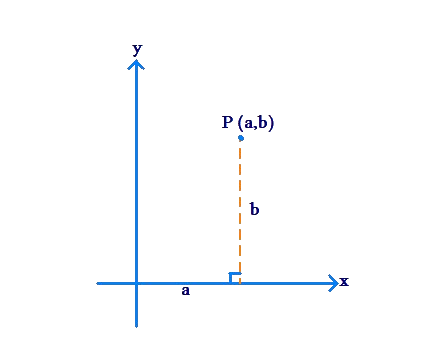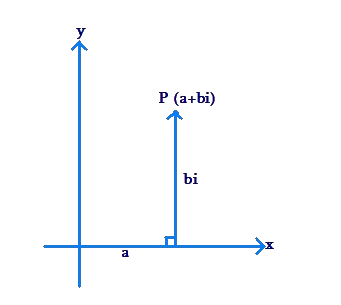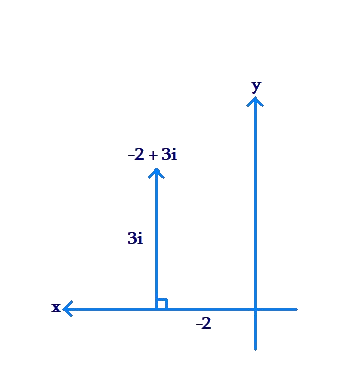# A Complex Number as a Point in the Plane

A Complex Number as a Point in the Plane

In our discussion on Real Numbers, we saw that every point on a straight number line can be represented using a real number, and every real number corresponds to a point on the real number line. In other words, the Real set enables us to label points on a line with unique number representations.

Is it possible to label points in a plane using numbers? In Coordinate Geometry, we have studied that this is possible using a pair of numbers: an x-coordinate and a y-coordinate. The position of any point in the plane can be uniquely specified using these two coordinates:In the language of Complex Numbers, the point .$$P\left( {a,b} \right)$$. can be represented as the number $$a + bi$$. Let us examine this entity $$a + bi$$ in more detail.

• The number $$a + bi$$ is a sum, or complex, of two entities: a and bi (hence the name complex numbers)

• The first entity, a, is a real number. It tells us the x-coordinate of the point P.

• The second entity, bi (or $$b \times i$$), is a non-real number, in the sense that it does not lie in the Real set. You should interpret bi as “b units along the i direction”, where the i direction is perpendicular to the real line.

Thus, to reach the point $$P\left( {a + bi} \right)$$, you need to move a units in the real direction (along the real line), and b units in the i direction (perpendicular to the real line):For example, let us plot the point corresponding to the number $$- 2 + 3i$$ in the plane. We move $$- 2$$ units along the real direction, and 3 units along the i-direction:Thus, every point in the plane can be represented using a number of the form $$x + iy$$. Conversely, every number of the form $$x + iy$$ corresponds to a point in the plane.

In other words, just like the Real Set enables us to label every point on the Real Line, the Complex Set enables us to label every point in the Complex Plane:

• Real Set $$\Leftrightarrow$$ Real Line

• Complex Set $$\Leftrightarrow$$ Complex Plane

More Important Topics
Numbers
Algebra
Geometry
Measurement
Money
Data
Trigonometry
Calculus